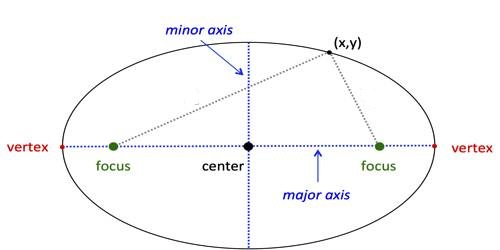Ellipse in Coordinate Geometry

Co-ordinates Geometry is a system of geometry where the position of points on the plane is described using an ordered pair of numbers. Straight lines in coordinate geometry are the same idea as in regular geometry, except that they are drawn on a coordinate plane and we can do more with them.

An ellipse can be defined as the locus of all points that satisfy the equation. It is the locus of all points of the plane whose distances to two fixed points add to the same constant.

x2/a+ y2/b= 1

where; x, y are the coordinates of any point on the ellipse; a, b is the radius on the x and y-axes respectively.Ellipse:

(i) Standard equation of ellipse is: x2/a2 + y2/b2 = 1 ……….(1)

(a) Its centre is the origin and major and minor axes are along x and y-axes respectively ; length of major axis = 2a and that of minor axis = 2b and eccentricity = e = √[1 – (b2/a2)]

(b) If S and S’ be the two foci and P (x, y) any point on it then SP = a – ex, S’P = a + ex and SP + S’P = 2a.

(c) The point (x1, y1) lies outside, on or inside the ellipse (1) according as x12/a2 + y12/b2 – 1 > , = or < 0.

(d) The parametric equations of the ellipse (1) are x = a cos θ, y = b sin θ where θ is the eccentric angle of the point P (x, y) on the ellipse (1); (a cos θ, b sin θ) are called the parametric co-ordinates of P.

(e) The equation of auxiliary circle of the ellipse (1) is x2 + y2 = a2.

(ii) Other forms of the equations of ellipse:

(a) x2/a2 + y2/b2 = 1. Its centre is at the origin and the major and minor axes are along y and x-axes respectively.

(b) [(x – α)2]/a2 + [(y – β)2]/b2 = 1.

The center of this ellipse is at (α, β) and the major and minor ones are parallel to x-axis and y-axis respectively.
Information Source: